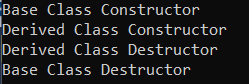# Virtual Destructors in C++

## Virtual Destructors in C++ with Examples:

In this article, I am going to discuss Virtual Destructors in C++ with Examples. Please read our previous article where we discussed Destructors in C++ with Examples. At the end of this article, we will learn the following pointers,

1. Destructors in C++
2. Destructor in Inheritance
3. Virtual Destructors in C++
4. Pure Virtual Destructors in C++
##### Destructors in C++:

A destructor is a special member function of a class that is used for releasing the resources acquired by the object of a class. Suppose in a class, if you are using some file, so in the constructor, you will open the file and, in the destructor, you will close the file. If a class is using some extra memory in the heap, it may be acquired in the constructor or in some other function also. But when the object is destroyed, it should release the memory.

Another example is a class that may be using a network connection. In the constructor, it may be getting the network connection. And in destructor, it should release the network connection. It is just like, at your college, in your sports room or sports department, you want to play a sport. So, you will take all the things that are required. You acquire them, then you play and when you have finished your game, you will return those things. That’s what is in programming also. If we acquire resources, we should release them. Usually, we acquire resources in constructors, and in destructors we release them. Even if you are acquiring resources at any other place but destructor is the right place for releasing the resources. So that’s the use of destructors.

##### Example to Understand Destructors in C++:

Let us understand Destructors in C++ with one example. For a better understanding, please have a look at the following example.

```#include <iostream>
using namespace std;
class Base
{
public:
Base ()
{
cout << "Base Class Constructor" << endl;
}
~Base ()
{
cout << "Base Class Destructor" << endl;
}
};

class Derived:public Base
{
public:
Derived ()
{
cout << "Derived Class Constructor" << endl;
}
~Derived ()
{
cout << "Derived Class Destructor" << endl;
}
};

int main()
{
Derived d;
return 0;
}
```
###### Output: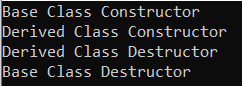Here, we have a Base class. It is having two public members functions that are Base() and ~Base() i.e. one public constructor and one public destructor. Constructor and Destructor both will have the same name as the class name but the destructor function is preceded with the tilde (~) symbol.

Next, we have a Derived class which is inherited from the Base class. It is also having two public member functions that are constructor and destructor. Now let us understand what means by destructor and when it is called.

Inside the main function, we have created an object d of the Derived class. We have already learned about the constructors. So, we know how the constructors will be called when an object of the derived class is created. So, the first constructor call is of the constructor of the Base class. So first, “Base Class Constructor” will be printed on the screen. Then after this, the derived class constructor will be called. So “Derived Class Constructor” will be printed on the screen.

##### C++ Destructor in Inheritance:

So, when the destructor will be called? A destructor is called when the object is being destroyed. So, when the object is constructed, the constructor is called and when the object is destroyed, the destructor is called. When the object is getting destroyed? Once the main function ends, the object will be destroyed because the program itself ends. So, the object will no more present in the memory, it will be destroyed.

##### How the Destructors are Called in C++?

First, the destructor of the Derived class will be called then the destructor of the Base class will be called. The below messages will be printed on the screen.

Derived Class Destructor
Base Class Destructor

So that’s it. This is how Destructors in C# are called in inheritance. So, if you are creating an object of a derived class and when it is being destroyed, first, the destructor of the Derived class will be called then the destructor of the Base class will be called. So, in contrast to the constructor, destructors are called from the bottom-up approach.

##### Virtual Destructors in C++:

A Virtual Destructor in C++ is used to release the memory space which is allocated by the derived class object while deleting the object of the derived class using a base class pointer object.

Let us understand Virtual Destructors in C++ with an Example. Please have a look at the below example. This is the same example as the previous one, only changes in the main method.

```#include <iostream>
using namespace std;
class Base
{
public:
Base ()
{
cout << "Base Class Constructor" << endl;
}
~Base ()
{
cout << "Base Class Destructor" << endl;
}
};

class Derived:public Base
{
public:
Derived ()
{
cout << "Derived Class Constructor" << endl;
}
~Derived ()
{
cout << "Derived Class Destructor" << endl;
}
};

int main()
{
Base *p = new Derived();
delete p;
return 0;
}
```

Here, inside the Main method, we have a Base class pointer and the object is of the Derived class. That means the base class pointer is pointing to the derived class object. We have already discussed these things in our previous articles. We are creating it dynamically. This is created inside the heap. Then on the next line, we are deleting p because dynamically created memory should be deleted when not required using the delete operator. So new Derived object will be destroyed.

Here, the object we created is of the Derived class. And when the object is deleted or destroyed then which function will be called? The Destructor function will be called. But whose destructor function will be called? You might be thinking that the pointer p was pointing to the Derived class object, so first Derived class destructor will be called, and then the Base class destructor function will be called. But no, that will not happen.

Which constructor will be called? Remember in C++, the function calls are depending upon the pointer, not upon the object. So, the pointer p is of the Base class. So only the Base class destructor will be called. The derived class destructor will not be called. So, when you run the above example code, you will get the following output.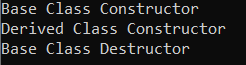But suppose you want the destructor of the derived class should also be called and then the Base class destructor called. If you want to do this, then you have to write virtual keyword before the name of Base class Destructor as,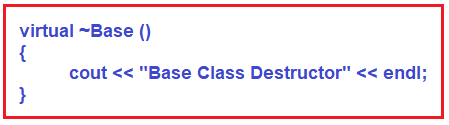If we write virtual keyword there, then even though the pointer is of Base class and object is of Derived class and when the object is deleted then first, the destructor of Derived class will be called and then the destructor of Base class will be called. The complete example code is given below.

```#include <iostream>
using namespace std;
class Base
{
public:
Base ()
{
cout << "Base Class Constructor" << endl;
}
virtual ~Base ()
{
cout << "Base Class Destructor" << endl;
}
};

class Derived:public Base
{
public:
Derived ()
{
cout << "Derived Class Constructor" << endl;
}
~Derived ()
{
cout << "Derived Class Destructor" << endl;
}
};

int main()
{
Base *p = new Derived();
delete p;
return 0;
}
```
###### Output: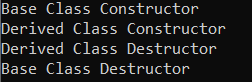So, this is how virtual destructors work. If you don’t make the Base class destructor virtual and you are using a pointer of the Base class and object of the Derived class then only the resources acquired by the Base class code will be released. But the resources acquired by Derived class code will not be released because that will not execute. So, this must also execute. If you are using the Base class pointer and Derived class object then you should make the Base class destructor virtual. This is useful for runtime polymorphism. If you want to achieve runtime polymorphism then you can write like this. So that’s it. This is all about Virtual Destructors in C++.

##### Pure Virtual Destructors in C++

It is also possible in C++ to declare the destructor as a Pure Virtual Destructor. The only difference between Virtual Destructor and Pure Virtual Destructor in C++ is that the pure virtual destructor will make the class Abstract, hence we cannot create an object of that class but we can create a pointer of that class. There is no requirement to implement pure virtual destructors in the derived classes as we are going to create instances of the derived which are going to be pointed by the Base class pointer.

For a better understanding, please have a look at the below example. Here, we are declaring the Base class destructor as pure virtual, and then outside the Base class, we are providing the body of the Pure Virtual Destructor using the class name i.e. Base and scope resolution operator. Once we declared the destructor as Pure Virtual, so we cannot create an instance of the Base class, we can only create a pointer of the Base class which will point to the Derived class object.

```#include <iostream>
using namespace std;
class Base
{
public:
Base ()
{
cout << "Base Class Constructor" << endl;
}
//Pure Virtual Destructor
virtual ~Base () = 0;
};
// Definition of Pure Virtual Destructor
Base::~Base()
{
cout << "Base Class Destructor" << endl;
}
class Derived:public Base
{
public:
Derived ()
{
cout << "Derived Class Constructor" << endl;
}
~Derived ()
{
cout << "Derived Class Destructor" << endl;
}
};

int main()
{
//We cannot Create an instance of Base Class
//Base *b = new Base();
Base *p = new Derived();
delete p;
return 0;
}
```
###### Output: